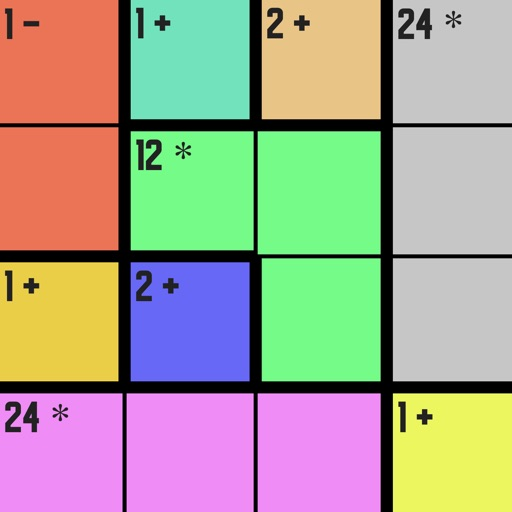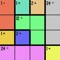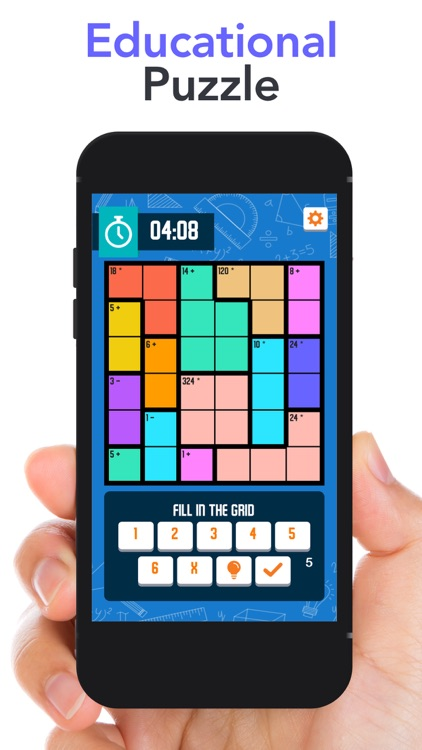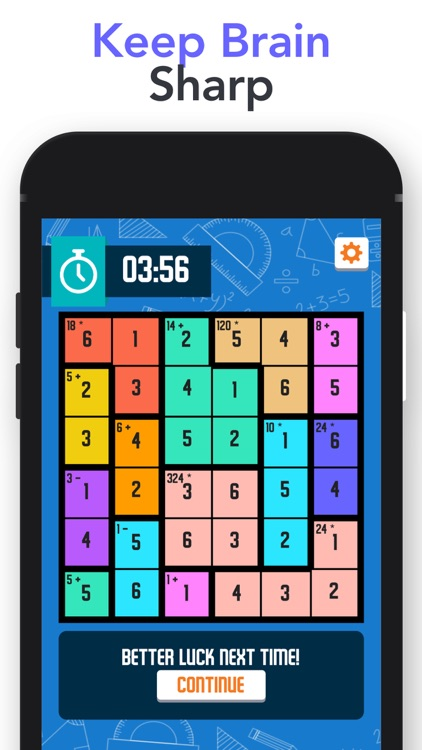## Sudoku is a game where you have to fill in the grid so that every row and every column contains the digits# Calcudoka – sudoku solver gameSudoku is a game where you have to fill in the grid so that every row and every column contains the digits. Each box must also be calculated according to number and operator, there is only 1 solution for each puzzle.Version
1.1
Rating
NA
Size
19Mb
Genre
Board Puzzle
Last updated
February 6, 2020
Release date
October 31, 2019

### App Store Description

Sudoku is a game where you have to fill in the grid so that every row and every column contains the digits. Each box must also be calculated according to number and operator, there is only 1 solution for each puzzle.

How to play:
1. The objective is to fill the grid in with the digits 1 to 3 (depends on the grid size)
2. Each row contains exactly one of each digit
3. Each column contains exactly one of each digit
4. Each bold-outlined group of cells is a cage containing digits which achieve the specified result using the specified mathematical operation: addition (+), subtraction (−), multiplication (×), and division (÷).

Features:
4 different difficulty auto generated puzzle to play (Easy, Normal, Hard, Expert)

SUBSCRIPTION PRICING AND TERMS

Subscription Options: